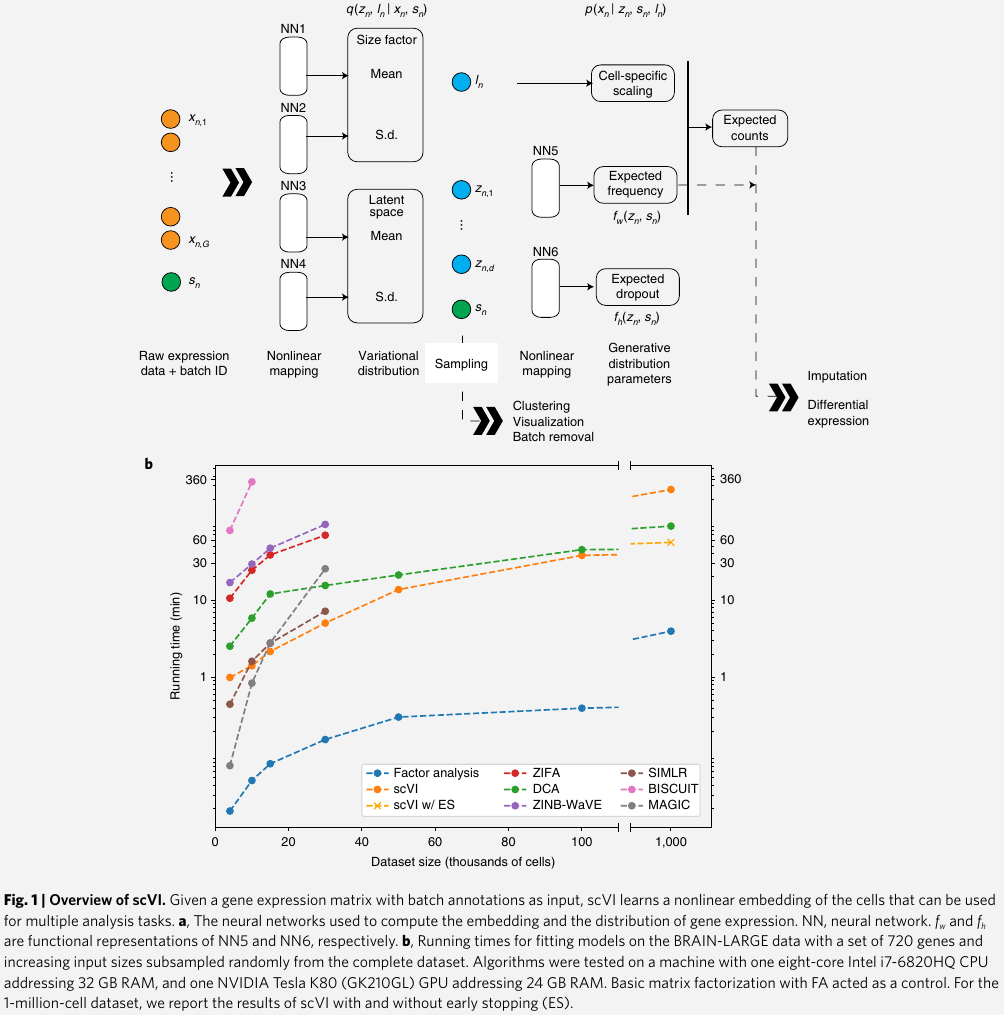# Deep Generative Modeling for Single-cell Transcriptomics

##### Posted on Jun 29, 2023model the observed expression $x_{ng}$ of each gene $g$ in each cell $n$ as a sample drawn from a zero-inflated negative binominal (ZINB) distribution $p(x_{ng}\mid z_n, s_n, \ell_n)$

• $s_n$: batch annotation of each cell (if available)
• $z_n,\ell_n$: unobserved random variables
• $\ell_n$: one-dimensional Gaussian that represents nuisance variation due to differences in capture efficiency and sequencing depth, and serves as a cell-specific scaling factor
• $z_n$: low-dimensional vector of Gaussian representing the remaining variation, which should better reflect biological differences between cells. use it to represent each cell as a point in a low-dimensional latent space that served for visualization and clustering

a neural network maps the latent variables to the parameters of the ZINB distribution. This mapping goes through intermediate values $\rho_g^n$, which provide a batch-corrected, normalized estimate of the percentage of transcripts in each cell $n$ that originate from each gene $g$

use these estimates for differential expression analysis and its scaled version (multiplying $\rho_g^n$ by the estimated library size $\ell_n$) for imputation

derive an approximation for the posterior distribution of the latent variables $q(z_n,\log \ell_n\mid x_n, s_n)$ by training another neural network using variational inference and a scalable stochastic optimization procedure.

Published in categories Note